## 大众Polo Plus，配置太低，买它靠情怀？

2019年07月04日 18:20 来源：车行天下 超过：14660次关注

中国市场其实是没有什么好的合资小型车的。因为小型车受制于成本的原因，通常价格没有办法卖得很高，所以对于合资品牌来说利润太低了。目前市面上的小型车，主要是自主品牌在研发和生产，合资品牌是不涉足的，我们现在能够脱口而出的合资小型车，除了现代和起亚这样的合资品牌以外，就是大众Polo Plus和本田飞度了，这两款车是目前市面上关注度最高的，而且是品质非常好的合资小型车。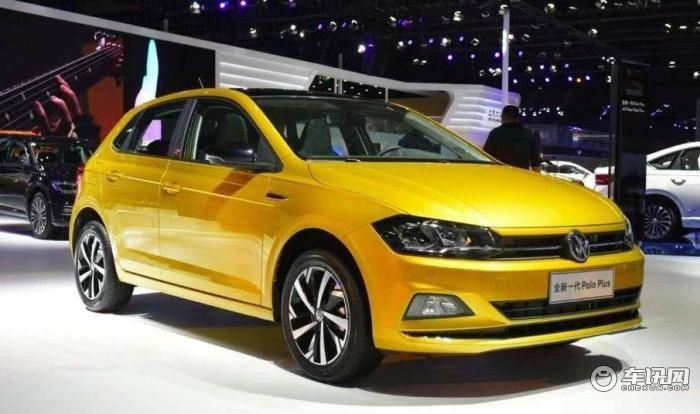随着大众Polo的换代，在市场上引起了非常大的热议，因为这款车价格实在是太贵了，作为一个小型车，大众Polo Plus的价格是9.99~12.59万元。最低配的9.99万元是一款手动挡的车型，并不是走量的车型，而自动挡的车型最低配也要10.99万元起，接近11万的价格了。而11万的价格消费者能够买到什么配置呢?可以说是非常非常寒酸的。因此它也就成了目前市面上性价比最差的合资小型车。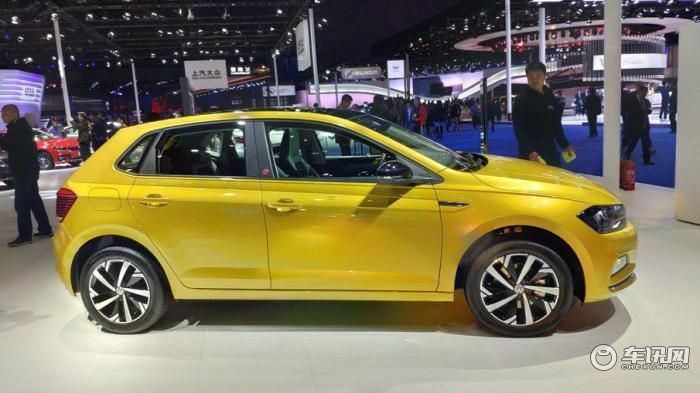不过由于大众Polo Plus的定位以及它时尚的造型设计，还是能够受到很多一线城市，年轻消费者的青睐，满足他们城市代步的基本需求。不过付出的代价有点大，因为相同的价格可以买到更好的紧凑型车。而如果消费者喜欢Polo Plus这样尺寸不大的车，就不得不接受它昂贵的价格。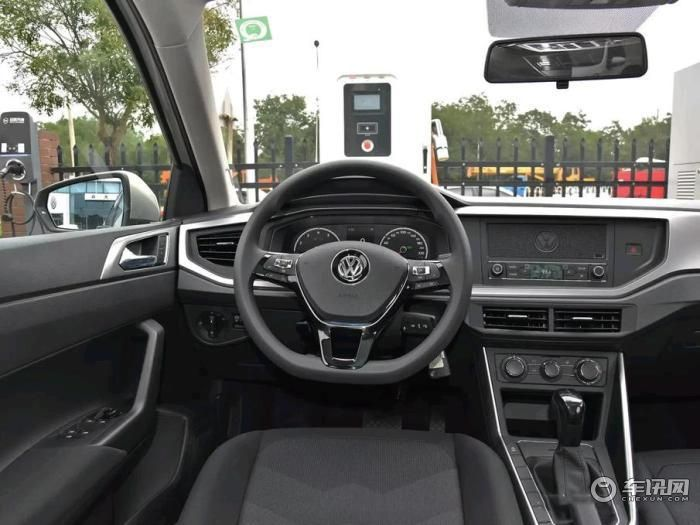6.5寸中控屏幕，11.59万起

大众Polo Plus在中低配车型上使用的是6.5英寸的中控屏幕，但是要11.59万元起的价格才可以买到。其实由于大众MQB平台的通用性，中控大屏幕升级的成本也很低，大概也就五六百块钱，不过对于厂商来说500块钱就是很大的一个成本了，大家可以想一下，如果一年卖10万辆，每辆车500块钱成本，那么10万辆就是5000万元。

但是对于消费者来说实在难以接受，就是11.59万元买不了一个带中控屏的车。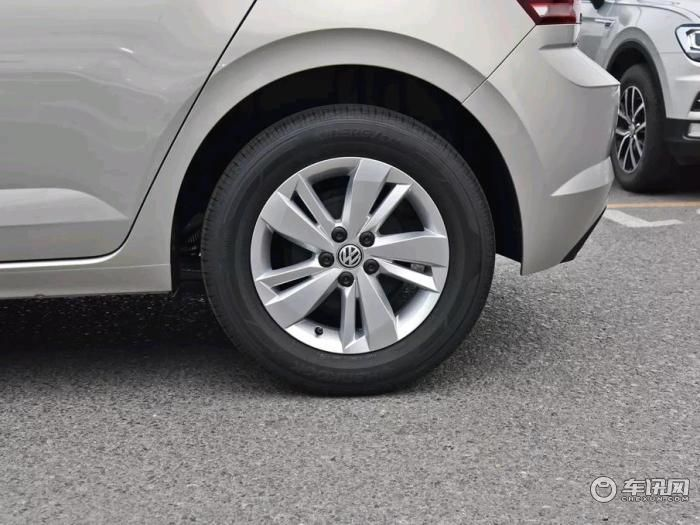后轮盘式刹车，14.39万起

鼓式刹车和盘式刹车，是有很明显的区别的，两者并没有好坏之分，通常载重量比较大的车用鼓式刹车，因为它的制动效果好，但缺点是有热衰减而且不防水，通常对于家用轿车来说，用盘式刹车是最为普遍的。因为家用轿车重量轻，在同样的制动效果的前提下，盘式刹车有更好的散热效果，而且不怕水。

从成本上，鼓式刹车成本低，而Polo Plus只有顶配车型上售价14.39万元的版本上使用了盘式刹车。可以说是很抠门了。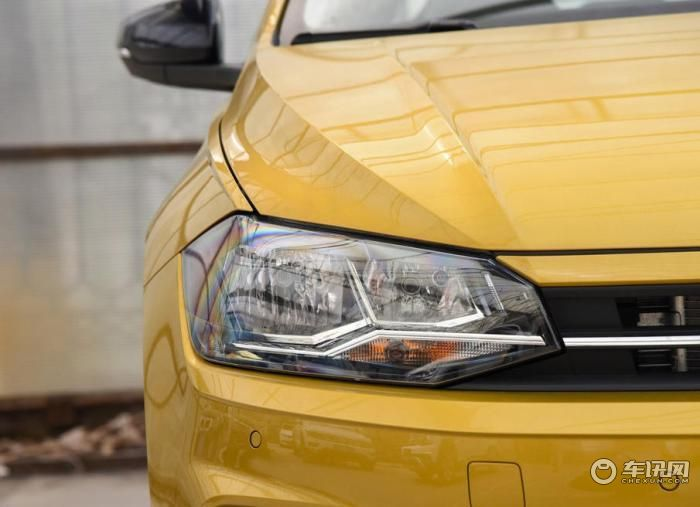LED大灯，全系没有，顶配可以选装

目前在国产轿车上使用LED灯已经非常非常普遍了，因为它的亮度更高，而且更加的节能，使用寿命也更长。但是Polo Plus全系都是卤素大灯，只有最顶配的车型，消费者才有权利选装LED大灯。如果你买的不是顶配，那你选装也没有办法选，只能去后期自己改装，而改装的成本，其实也并不高，在电商平台上花几千块钱就可以升级成LED大灯了。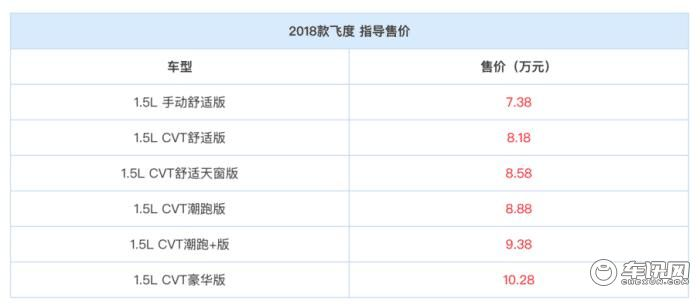Polo Plus的高价是给自己留下了降价区间?

很多人说Polo Plus的高价格，是给自己留下了降价空间。其实我并不这么认为，因为我们看一下飞度，相比起大众来说，飞度的价格可以说是非常厚道的。起步价是7.38万元，最顶配的价格是10.28万元。而且目前市面上飞度也有一些优惠，所以我并不认为Polo Plus的高价格是给自己留下的降价空间，而是厂商为了赚更多的利润，故意的把价格定得非常高，本来也没想卖的便宜。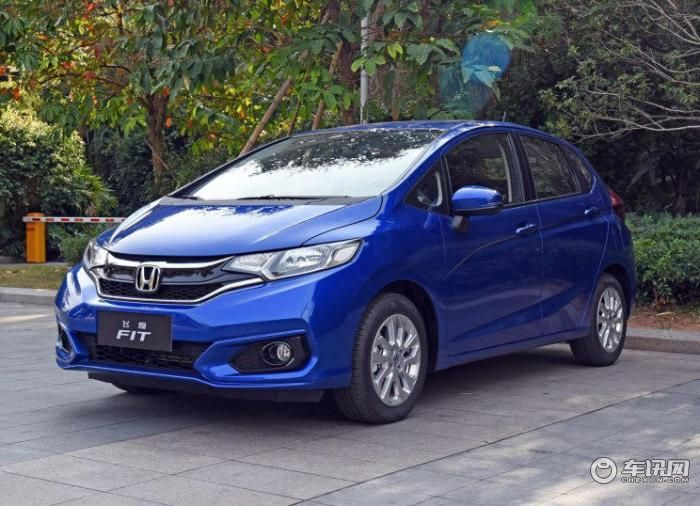同级别的合资品牌车型配置不如自主品牌的车型高，是可以理解的，因为自主品牌一直是以配置的丰富程度来吸引消费者的，不过Polo Plus是一款小型车的定位，而且还卖十几万的价格，配置还如此之低，真的是目前车市比较罕见的情况。所以也更加凸显了飞度的性价比优势。

0-500 字已有评论 0条 查看评论>>

### 热门标签

﻿
• 快速找车
• 选择品牌
• 选择品牌
• A  奥迪
• A  阿斯顿·马丁
• A  阿尔法·罗密欧
• B  宝沃
• B  布加迪
• B  巴博斯
• B  保时捷
• B  宾利
• B  奔驰
• B  宝马
• B  本田
• B  别克
• B  标致
• B  比亚迪
• B  宝骏
• B  北汽制造
• B  北汽新能源
• B  北汽幻速
• B  北汽威旺
• B  北京汽车
• B  奔腾
• B  北汽绅宝
• C  长安
• C  长安商用
• C  长城
• C  昌河
• D  大众
• D  道奇
• D  DS
• D  东南
• D  东风风神
• D  东风风行
• D  东风小康
• D  东风风度
• D  东风
• F  福特
• F  丰田
• F  菲亚特
• F  法拉利
• F  福田
• F  福迪
• F  福汽启腾
• G  观致
• G  广汽传祺
• G  广汽吉奥
• G  GMC
• H  红旗
• H  汉腾汽车
• H  哈弗
• H  哈飞
• H  海格
• H  海马
• H  华颂
• H  黄海
• H  华泰
• H  恒天
• J  吉利汽车
• J  捷豹
• J  Jeep
• J  江淮
• J  江铃
• J  金杯
• J  九龙
• J  金旅
• K  凯翼
• K  凯迪拉克
• K  克莱斯勒
• K  科尼塞克
• K  卡威
• K  开瑞
• L  路虎
• L  林肯
• L  劳斯莱斯
• L  兰博基尼
• L  雷克萨斯
• L  铃木
• L  雷诺
• L  理念
• L  力帆
• L  莲花汽车
• L  猎豹
• L  路特斯
• L  陆风
• M  马自达
• M  MG
• M  MINI
• M  玛莎拉蒂
• M  摩根
• M  迈凯轮
• N  纳智捷
• O  欧宝
• O  讴歌
• O  欧朗
• Q  奇瑞
• Q  起亚
• Q  启辰
• R  日产
• R  荣威
• R  瑞麒
• S  三菱
• S  斯威汽车
• S  萨博
• S  smart
• S  斯柯达
• S  斯巴鲁
• S  思铭
• S  双龙
• S  上汽大通
• S  双环
• T  特斯拉
• T  腾势
• W  沃尔沃
• W  五菱汽车
• W  五十铃
• W  威兹曼
• W  威麟
• X  现代
• X  雪佛兰
• X  雪铁龙
• X  西雅特
• Y  一汽
• Y  英菲尼迪
• Y  英致
• Y  依维柯
• Y  野马汽车
• Y  永源
• Z  众泰
• Z  中华
• Z  中兴
• Z  知豆
• 选择车系
• 选择车系
• 车型对比
• 选择品牌
• 选择品牌
• A  奥迪
• A  阿斯顿·马丁
• A  阿尔法·罗密欧
• B  宝沃
• B  布加迪
• B  巴博斯
• B  保时捷
• B  宾利
• B  奔驰
• B  宝马
• B  本田
• B  别克
• B  标致
• B  比亚迪
• B  宝骏
• B  北汽制造
• B  北汽新能源
• B  北汽幻速
• B  北汽威旺
• B  北京汽车
• B  奔腾
• B  北汽绅宝
• C  长安
• C  长安商用
• C  长城
• C  昌河
• D  大众
• D  道奇
• D  DS
• D  东南
• D  东风风神
• D  东风风行
• D  东风小康
• D  东风风度
• D  东风
• F  福特
• F  丰田
• F  菲亚特
• F  法拉利
• F  福田
• F  福迪
• F  福汽启腾
• G  观致
• G  广汽传祺
• G  广汽吉奥
• G  GMC
• H  红旗
• H  汉腾汽车
• H  哈弗
• H  哈飞
• H  海格
• H  海马
• H  华颂
• H  黄海
• H  华泰
• H  恒天
• J  吉利汽车
• J  捷豹
• J  Jeep
• J  江淮
• J  江铃
• J  金杯
• J  九龙
• J  金旅
• K  凯翼
• K  凯迪拉克
• K  克莱斯勒
• K  科尼塞克
• K  卡威
• K  开瑞
• L  路虎
• L  林肯
• L  劳斯莱斯
• L  兰博基尼
• L  雷克萨斯
• L  铃木
• L  雷诺
• L  理念
• L  力帆
• L  莲花汽车
• L  猎豹
• L  路特斯
• L  陆风
• M  马自达
• M  MG
• M  MINI
• M  玛莎拉蒂
• M  摩根
• M  迈凯轮
• N  纳智捷
• O  欧宝
• O  讴歌
• O  欧朗
• Q  奇瑞
• Q  起亚
• Q  启辰
• R  日产
• R  荣威
• R  瑞麒
• S  三菱
• S  斯威汽车
• S  萨博
• S  smart
• S  斯柯达
• S  斯巴鲁
• S  思铭
• S  双龙
• S  上汽大通
• S  双环
• T  特斯拉
• T  腾势
• W  沃尔沃
• W  五菱汽车
• W  五十铃
• W  威兹曼
• W  威麟
• X  现代
• X  雪佛兰
• X  雪铁龙
• X  西雅特
• Y  一汽
• Y  英菲尼迪
• Y  英致
• Y  依维柯
• Y  野马汽车
• Y  永源
• Z  众泰
• Z  中华
• Z  中兴
• Z  知豆
• 选择车系
• 选择车系
• 选择车型
• 选择车型
• 意见反馈